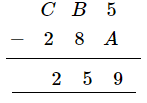# Replace A, B, C by suitable numerals.

Question:

Replace A, B, C by suitable numerals.Solution:

$(\mathrm{B} \times 3)=\mathrm{B}$

Then, B can either be 0 or 5 .

If $\mathrm{B}$ is 5 , then 1 will be carried.

Then, $\mathrm{A} \times 3+1=\mathrm{A}$ will not be possible for any number.

$\therefore \mathrm{B}=0$

$\mathrm{A} \times 3=\mathrm{A}$ is possible for either 0 or 5 .

If we take $\mathrm{A}=0$, then all number will become 0 . However, this is not possible.

$\therefore \mathrm{A}=5$

Then, 1 will be carried.

$\therefore \mathrm{C}=1$

$\therefore \mathrm{A}=5, \mathrm{~B}=0$ and $\mathrm{C}=1$